# CAT 2017 – Slot 2 – Quantitative Ability – The base of a vertical pillar with uniform cross section is a trapezium

Q. 1: The base of a vertical pillar with uniform cross section is a trapezium whose parallel sides are of lengths 10 cm and 20 cm while the other two sides are of equal length. The perpendicular distance between the parallel sides of the trapezium is 12 cm. If the height of the pillar is 20 cm, then the total area, in sq cm, of all six surfaces of the pillar is
A) 1300
B) 1340
C) 1480
D) 1520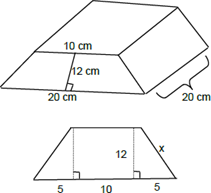Given, the non-parallel sides are equal. Let the non-parallel sides be x cm each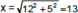So, we have 6 faces, two are trapezoid faces and 4 are rectangular faces. Area of 2 trapeziums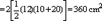Area of 4 rectangles

= 2[13 × 20] + 20(20) + 10(20) = 1120 cm2

Total area = 1120 + 360 = 1480 cm2 Choice (3)

##### Q. 2: The points (2, 5) and (6, 3) are two end points of a diagonal of a rectangle. If the other diagonal has the equation y = 3x + c, then c isA) -5 B) -6 C) -7 D) -8

Explanation:- In a rectangle, diagonals bisect each other, so one diagonal should pass through the midpoint of the other. Midpoint of the diagonal connecting (2, 5) and (6, 3)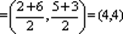The other diagonal, y = 3x + c should also pass through (4, 4). On substitution, 4 = 3 (4) + c ⇒ –8.

Q. 3: ABCD is a quadrilateral inscribed in a circle with centre O. If ∠COD = 120 degrees and ∠BAC = 30 degrees, then the value of ∠BCD (in degrees) is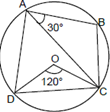Explanation:-

Given, ∠COD = 120° and ∠BAC = 30°.

As ∠COD = 120°, ∠DAC = 60° (Central angle)

∠DAC + ∠BAC = 60° + 30° = 90°

∠A = 90° ⇒ ∠BCD = 90° (Opposite angles are supplementary in a cyclic quadrilateral)

Q. 4: If three sides of a rectangular park have a total length 400 ft, then the area of the park is maximum when the length (in ft) of its longer side is

Explanation:- Let one side be l and the other be b. (l is not necessarily greater than b) Given, 2l + b = 400

For area to be maximum, lb should be maximum.

∴ l (400 – 2l) should be maximum

l (400 – 2l) = l (2) (200–l) = 2 (l) (200 – l)

l (200 – l) will be maximum when l = 200 – l or 2l = 200

⇒ l = 100

If l = 100, b = 200.

∴ The longer side must be 200 feet long.

Q. 5: Let P be an interior point of a right-angled isosceles triangle ABC with hypotenuse AB. If the perpendicular distance of P from each of AB, BC, and CA is 4 (√2 – l) cm, then the area, in sq cm, of the triangle ABC is

Explanation:-    As P is equidistant from the sides, P is the in center of the triangle. r is the in radius of the triangle, viz. 4(√2 – 1) cm

Let the sides of the triangle be a, a, a √2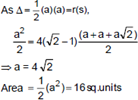##### Checkout Other Questions of CAT 2017 Slot 2 Paper:

Verbal Ability :              |   Q.01- Q.06  |  Q.07- Q.12  |  Q.13- Q.18  |  Q.19- Q.21  |  Q.22- Q.24  |  Q.25- Q.29  |  Q.30 – Q.34  |

Logical Reasoning :    |   Q.29 – Q.32  |

Quantitative Aptitude: |

### Free Material Area